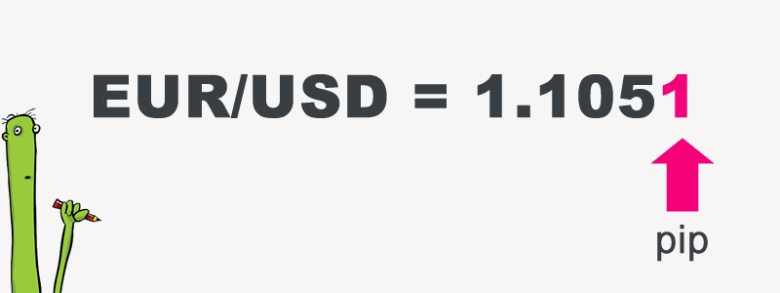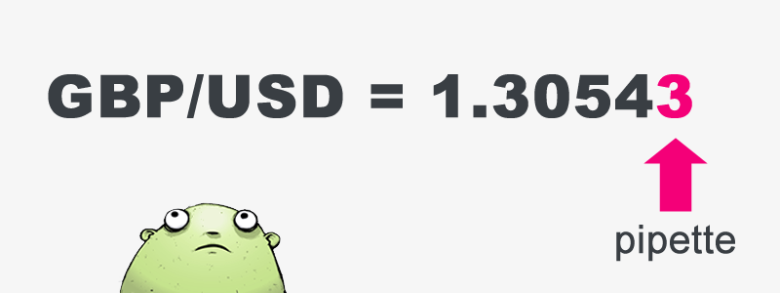Here is where we’re going to do a little math. You’ve probably heard of the terms “pips,” “pipettes,” and “lots” thrown around, and here we’re going to explain what they are and show you how their values are calculated.

Take your time with this information, as it is required knowledge for all forex traders.

Don’t even think about trading until you are comfortable with pip values and calculating profit and loss.

## What the heck is a Pip?

The unit of measurement to express the change in value between two currencies is called a “pip.”

If EUR/USD moves from 1.1050 to 1.1051, that .0001 USD rise in value is ONE PIP.

A pip is usually the last decimal place of a quotation.

Most pairs go out to 4 decimal places, but there are some exceptions like Japanese Yen pairs (they go out to two decimal places).## What is a Pipette?

There are brokers that quote currency pairs beyond the standard “4 and 2” decimal places to “5 and 3” decimal places.

They are quoting FRACTIONAL PIPS, also called “pipettes.”

For instance, if GBP/USD moves from 1.30542 to 1.30543, that .00001 USD move higher is ONE PIPETTE.## How to Calculate the Value of a Pip

As each currency has its own relative value, it’s necessary to calculate the value of a pip for that particular currency pair.

In the following example, we will use a quote with 4 decimal places.

For the purpose of better explaining the calculations, exchange rates will be expressed as a ratio (i.e., EUR/USD at 1.2500 will be written as “1 EUR / 1.2500 USD”)

### Example #1: USD/CAD = 1.0200

(The value change in counter currency) times the exchange rate ratio = pip value (in terms of the base currency)

Or simply as:

Using this example, if we traded 10,000 units of USD/CAD, then a one pip change to the exchange rate would be approximately a 0.98 USD change in the position value (10,000 units x 0.0000984 USD/unit).

We say “approximately” because as the exchange rate changes, so does the value of each pip move.

### Example #2: GBP/JPY = 123.00

Here’s another example using a currency pair with the Japanese Yen as the counter currency.

Notice that this currency pair only goes to two decimal places to measure a 1 pip change in value (most of the other currencies have four decimal places). In this case, a one pip move would be .01 JPY.

(The value change in counter currency) times the exchange rate ratio = pip value (in terms of the base currency)

[.01 JPY] x [1 GBP/123.00 JPY]

Or simply as:

[(.01 JPY) / (123.00 JPY)] x 1 GBP = 0.0000813 GBP

So, when trading 10,000 units of GBP/JPY, each pip change in value is worth approximately 0.813 GBP.

## Finding the Pip Value in your Account Denomination

Now, the final question to ask when figuring out the pip value of your position is, “what is the pip value in terms of my account currency?”

After all, it is a global market and not everyone has their account denominated in the same currency.

This means that the pip value will have to be translated to whatever currency our account may be traded in.

This calculation is probably the easiest of all; simply multiply/divide the “found pip value” by the exchange rate of your account currency and the currency in question.

If the “found pip value” currency is the same currency as the base currency in the exchange rate quote:

Using the GBP/JPY example above, let’s convert the found pip value of .813 GBP to the pip value in USD by using GBP/USD at 1.5590 as our exchange rate ratio.

If the currency you are converting to is the counter currency of the exchange rate, all you have to do is divide the “found pip value” by the corresponding exchange rate ratio:

.813 GBP per pip / (1 GBP/1.5590 USD)

Or

[(.813 GBP) / (1 GBP)] x (1.5590 USD) = 1.2674 USD per pip move

So, for every .01 pip move in GBP/JPY, the value of a 10,000 unit position changes by approximately 1.27 USD.

If the currency you are converting to is the base currency of the conversion exchange rate ratio, then multiply the “found pip value” by the conversion exchange rate ratio.

Using our USD/CAD example above, we want to find the pip value of .98 USD in New Zealand Dollars. We’ll use .7900 as our conversion exchange rate ratio:

0.98 USD per pip X (1 NZD/.7900 USD)

Or

[(0.98 USD) / (.7900 USD)] x (1 NZD) = 1.2405 NZD per pip move

For every .0001 pip move in USD/CAD from the example above, your 10,000 unit position changes in value by approximately 1.24 NZD.

Even though you’re now a math genius–at least with pip values–you’re probably rolling your eyes back and thinking, “Do I really need to work all this out?”

Well, the answer is a big fat NO. Nearly all forex brokers will work all this out for you automatically, but it’s always good for you to know how they work it out.

If your broker doesn’t happen to do this, don’t worry – you can use our Pip Value Calculator! Aren’t we awesome?

In the next lesson, we will discuss how these seemingly insignificant amounts can add up.